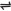## The Law of Mass Action and The Rate Equation

The law states that the rate of a chemical reaction is proportional to the active masses of each of the reactants at constant temperature.

The term Active Mass of a substance can be interpreted as the concentration of the substance raised to the appropriate power (which is its number of molecules written in the equation for that reaction).

Thus, the rate of a reaction is proportional to the concentrations (in mol/dm3) of the reactants in very dilute solutions.

For the hypothetical reaction:

aA + bBcC + dD

The rate of the forward reaction;

rf α [A]a [B]b ; rf = kf[A]a [B]b - (1)

The rate of the backward reaction;

rb α [C]c [D]d

rb = kb[C]c [D]d - (2)

kf and kb are called velocity or rate constant

At equilibrium rf = rb

Therefore, kf [A]a [B]b = kb [C]c [D]d - (3)

Note: the law of Mass Action enables one to express the conditions at equilibrium in terms of an equilibrium constant.

 Like This Post? Please Share!!!!!!!

Copyright , All Rights Reserved Free Chemistry Online | About Us | Usage of Content | Total Disclosures | Privacy Policy